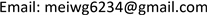﻿ 用于威布尔参数估计的高阶统计量 Higher Moment Statistics for Fitting Random Fatigue Life Data to Weibull Distribution Parameters

Statistics and Application
Vol.07 No.01(2018), Article ID:23949,7 pages
10.12677/SA.2018.71009

Higher Moment Statistics for Fitting Random Fatigue Life Data to Weibull Distribution Parameters

Guijin Wang

Alloy Steels Department, Central Iron & Steel Research Institute, BeijingReceived: Feb. 6th, 2018; accepted: Feb. 20th, 2018; published: Feb. 28th, 2018ABSTRACT

This paper presents a new approach to fitting fatigue datasets to three-parameter Weibull distribution by adjusting location parameter to and maximum accumulated fatigue life rate 99% in order to match the intrinsic higher moment statistics such as skewness and kurtosis from sample fatigue data directly. Thereafter, the parameters κ and λ of the Weibull distribution from various manufacture technology can be well estimated. The expected standard deviation σ, mean μ and accumulated failure life L10 can be calculated and compared as well. This new approach has been justified due to available experimental data of McCool.

Keywords:Weibull Distribution, Skewness, Kurtosis, Fitting Indicator η1. 引言

$f\left(t\right)=1-\mathrm{exp}\left\{-{\left(\frac{t-{t}_{o}}{\lambda }\right)}^{\kappa }\right\}\text{\hspace{0.17em}}\text{\hspace{0.17em}}\text{\hspace{0.17em}}\text{\hspace{0.17em}}\left(\lambda ,t-{t}_{o},\kappa \right)>0$ (1-1)

$f\left(t\right)=1-\mathrm{exp}\left\{-{\left(\frac{t}{\lambda }\right)}^{\kappa }\right\}\text{\hspace{0.17em}}\text{\hspace{0.17em}}\text{\hspace{0.17em}}\text{\hspace{0.17em}}\left(\lambda ,t,\kappa \right)>0$ (1-2)

$f\left(t\right)=1-\mathrm{exp}\left\{-{t}^{\kappa }\right\}\text{\hspace{0.17em}}\text{\hspace{0.17em}}\text{\hspace{0.17em}}\text{\hspace{0.17em}}\left(t,\kappa \right)>0$ (1-3)

2. 两参数威布尔分布的拟合

2.1. 极大似然法

$\frac{1}{\kappa }+\left[\left(\frac{1}{N}\right){\sum }_{i=1}^{N}\mathrm{ln}\left({t}_{i}\right)\right]-\left[{\sum }_{i=1}^{N}{t}_{i}^{k}\mathrm{ln}\left({t}_{i}\right)\right]/\left({\sum }_{i=1}^{N}{t}_{i}^{k}\right)=0$ (2)

$\lambda ={\left[\left(\frac{1}{N}\right){\sum }_{i=1}^{N}{t}_{i}^{k}\right]}^{1/\kappa }$ (3)

2.2. 威布尔分布的斜度和峭度

${\gamma }_{1}=\left[{\Gamma }_{3}-3{\Gamma }_{2}{\Gamma }_{1}+2{\Gamma }_{2}^{3}\right]/{\left[{\Gamma }_{2}-{\Gamma }_{1}^{2}\right]}^{3/2}$ (4)

${\gamma }_{2}=\left\{\left({\Gamma }_{4}-4{\Gamma }_{3}{\Gamma }_{1}+6{\Gamma }_{2}{\Gamma }_{1}^{2}-3{\Gamma }_{1}^{4}\right)/{\left({\Gamma }_{2}-{\Gamma }_{1}^{2}\right)}^{2}\right\}-3$ (5)

2.3. 拟合威布尔形状参数κ的具体步骤

$\eta =\left({\eta }_{1}+{\eta }_{2}\right)/2$ (6)

1) 先由小到大排列试样疲劳寿命值ti，用极大似然法算出两参数威布尔分布的形状参数 $\kappa$ 以及试样寿命的内禀斜度κ，峭度γ1及它们对应的形状参数 $\kappa \left({\gamma }_{1}\right)$$\kappa \left({\gamma }_{2}\right)$ 和拟合指数η。如果η偏离期望值1较远，则

2) 令位置参数to为非零值,调整to并重复步骤1)，逐步使η靠近1，

3) 如果η仍然偏离1较远，可同时调整to和tf重新计算η直到它接近最佳值1，这就得到拟合形状参数κ。

3.不同冶炼工艺下高温轴承钢的疲劳寿命

3.1. 试验设计

1982年McCool  发表了对三种高温轴承试验钢：M50，T15和高铬不锈钢分别采用真空自耗，真空冶炼 + 真空电弧炉除气或粉末冶金法。总共五种组合的寿命实验代码见表1

3.2. 试验结果及讨论

1) 原始数据的两参数威布尔分布拟合

t0，tf：疲劳失效开始时间和名义全失效时间(106转)；

κ，λ：极大似然法拟合的威布尔形状参数(无量纲)和尺寸参数(106转)；Table 1. Test codes for five manufacture technology

$\kappa \left({\gamma }_{1}\right)$$\kappa \left({\gamma }_{2}\right)$ ：试样数据内禀斜度和内禀峭度对应的威布尔形状参数；

μ和σ：试样寿命数据的均值和标准差(106转)；

δ2：拟合曲线相对于试样实验值的均方偏差(106转)2

${\delta }^{2}={\sum }_{i=1}^{9}{\left\{f\left({t}_{i}\right)-f\left({t}_{i,\text{weib}}\right)\right\}}^{2}/9$ (7)

CVEMM50： $f\left(t\right)=1-\mathrm{exp}\left\{{\left(t/7.03549\right)}^{2.30045}\right\}\text{\hspace{0.17em}}\text{\hspace{0.17em}}\text{\hspace{0.17em}}\text{\hspace{0.17em}}\text{\hspace{0.17em}}t>0,\text{\hspace{0.17em}}t={t}_{1},{t}_{2},\cdots ,{t}_{f}$

PP T15： $f\left(t\right)=1-\mathrm{exp}\left\{{\left(t/9.62393\right)}^{2.92675}\right\}\text{\hspace{0.17em}}\text{\hspace{0.17em}}\text{\hspace{0.17em}}\text{\hspace{0.17em}}\text{\hspace{0.17em}}t>0,\text{\hspace{0.17em}}t={t}_{1},{t}_{2},\cdots ,{t}_{f}$

VIMVAR M50： $f\left(t\right)=1-\mathrm{exp}\left\{{\left(t/11.353\right)}^{1.89622}\right\}\text{\hspace{0.17em}}\text{\hspace{0.17em}}\text{\hspace{0.17em}}\text{\hspace{0.17em}}\text{\hspace{0.17em}}t>0,\text{\hspace{0.17em}}t={t}_{1},{t}_{2},\cdots ,{t}_{f}$

PP M50： $f\left(t\right)=1-\mathrm{exp}\left\{{\left(t/11.862\right)}^{2.3971}\right\}\text{\hspace{0.17em}}\text{\hspace{0.17em}}\text{\hspace{0.17em}}\text{\hspace{0.17em}}\text{\hspace{0.17em}}t>0,\text{\hspace{0.17em}}t={t}_{1},{t}_{2},\cdots ,{t}_{f}$

PP CRB-7： $f\left(t\right)=1-\mathrm{exp}\left\{{\left(t/16.407\right)}^{3.4644}\right\}\text{\hspace{0.17em}}\text{\hspace{0.17em}}\text{\hspace{0.17em}}\text{\hspace{0.17em}}\text{\hspace{0.17em}}t>0,\text{\hspace{0.17em}}t={t}_{1},{t}_{2},\cdots ,{t}_{f}$

3.3. 数据经过to和tf修正后按斜度和峭度拟合Table 3. Fitting five fatigue dataset to two parameter Weibull distribution

NA*: No solution of k in normal range (0.5-5.0).Table 4. After correction of γ1 and γ2, fitting five datasets of fatigue life to three parameter Weibull distribution

CEVM M50： $f\left(t\right)=1-\mathrm{exp}\left\{{\left(\left(t-2.9359\right)/3.7341\right)}^{1.03187}\right\}\text{\hspace{0.17em}}\text{\hspace{0.17em}}\text{\hspace{0.17em}}\text{\hspace{0.17em}}t-{t}_{0}>0,\text{\hspace{0.17em}}t={t}_{1},{t}_{2},\cdots ,{t}_{f}$

VIMVARM50： $f\left(t\right)=1-\mathrm{exp}\left\{{\left(\left(t-5.46685\right)/4.0021\right)}^{1.0204}\right\}\text{\hspace{0.17em}}\text{\hspace{0.17em}}\text{\hspace{0.17em}}\text{\hspace{0.17em}}t-{t}_{0}>0,\text{\hspace{0.17em}}t={t}_{1},{t}_{2},\cdots ,{t}_{f}$

PP T15： $f\left(t\right)=1-\mathrm{exp}\left\{{\left(\left(t-3.2237\right)/6.81288\right)}^{1.08144}\right\}\text{\hspace{0.17em}}\text{\hspace{0.17em}}\text{\hspace{0.17em}}\text{\hspace{0.17em}}t-{t}_{0}>0,\text{\hspace{0.17em}}t={t}_{1},{t}_{2},\cdots ,{t}_{f}$

PP M50： $f\left(t\right)=1-\mathrm{exp}\left\{{\left(\left(t-2.66663\right)/10.279\right)}^{1.04561}\right\}\text{\hspace{0.17em}}\text{\hspace{0.17em}}\text{\hspace{0.17em}}\text{\hspace{0.17em}}t-{t}_{0}>0,\text{\hspace{0.17em}}t={t}_{1},{t}_{2},\cdots ,{t}_{f}$

PP CRB-7： $f\left(t\right)=1-\mathrm{exp}\left\{{\left(\left(t-6.2439\right)/10.786\right)}^{1.07474}\right\}\text{\hspace{0.17em}}\text{\hspace{0.17em}}\text{\hspace{0.17em}}\text{\hspace{0.17em}}t-{t}_{0}>0,\text{\hspace{0.17em}}t={t}_{1},{t}_{2},\cdots ,{t}_{f}$

3.4. 标准差σ，均值μ和额定寿命L10

$\sigma =\lambda {\left[\Gamma \left(1+2/\kappa \right)-{\Gamma }^{2}\left(1+1/\kappa \right)\right]}^{1/2}$ (8)

$\mu =\lambda \left(\Gamma \left(1+1/\kappa \right)\right)$ (9)Table 5. After correction of γ1 and γ2 fitting normalized five datasets of fatigue life to two parameter Weibull distributionTable 6. Standard variation, mean and life L10 from fitted Weibull two parameters and from five datasetsTable 7. Standard variation, mean and suggested life L10, from γ1γ2 corrected Weibull parameters and from dataset

4. 结论

1) 和现有威布尔参数拟合方法比较，通过调整位置参数to以及名义全失效时间tf，五种冶炼工艺下拟合三参数威布尔斜率κ相对集中，拟合指数η更接近。各个冶炼工艺的疲劳寿命分布的形状参数κ，尺寸参数λ，标准差σ，均值μ和额定寿命L10都比较合理。这是它优于现有拟合方法之处。但它也对寿命数据质量提出了更高要求。如果经过多次调整to和tf还得不到良好的拟合指数η，可能是样本数量不足，存在系统偏差或者疲劳数据服从其他分布所致。

2) 研究冶炼工艺对额定寿命L10的影响时，尺寸参数λ和形状参数κ都很重要。只有当不同数据组的κ值变化不大时，尺寸参数λ起更大作用。

3) 当需要了解在不同环境条件(诸如应力，温度，湿度，酸碱度等等)的额定寿命L10，最好按本文方法做系统的试验研究。

4) 本文只研究随机疲劳数据的拟合处理，未涉及失效微观机理，例如裂纹萌生及扩展的问题。本文结论只供参考。

Higher Moment Statistics for Fitting Random Fatigue Life Data to Weibull Distribution Parameters[J]. 统计学与应用, 2018, 07(01): 65-71. http://dx.doi.org/10.12677/SA.2018.71009

1. 1. Rhinne, H. (2009) The Weibull Distribution, A Handbook. CRC Press, New York.

2. 2. 王桂金. 拟合威布尔随机寿命S-N 曲线的新算法[J]. 统计学与应用, 2017, 6(2): 210-218.

3. 3. 王桂金. Weibull随机寿命的统计量[J]. 轴承, 2012(3): 38-42.

4. 4. McCool, J.J. (1982) Analysis of Sets of Two-Parameter Weibull Data Arising in Rolling Contact Endurance Testing. Rolling Contact Fatigue Testing of Bearing Steels, ASTM STP 771. Hoo, J. C. Ed., American Society of Testing and Materials, 293-319. https://doi.org/10.1520/STP36146S

5. 5. Zaretsky, E.V. (1988) Selection on Rolling Element Bearing Steels for Long-life Applications. Effect of Steel Manufacturing Processes on the Quality of Bearing Steels, ASTM STP 987, Philadelphia, 5-43. https://doi.org/10.1520/STP26225S